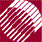ECE532 Biomedical Optics © 1998 Steven L. Jacques, Scott A. Prahl Oregon Graduate Institute

# Time-resolved Monte Carlo

## Hop/Drop photon to next position

The following sections (HOP/DROP, SPIN, CHECK) are contained within the HOP/DROP_SPIN_CHECK DO-WHILE loop. This loop propagates the photon until the maximum total pathlength (Lmax) is exceeded. Then the photon is labeled as "DEAD" = 0 and the DO-WHILE loop terminates. A new photon is launched by the RUN DO-WHILE loop.

```/* HOP/DROP_SPIN_CHECK DO-WHILE LOOP
*   Propagate one photon until it dies.
*******/
do {

/******************************************
*  Photon movement occurs within this HOP/DROP_SPIN_CHECK DO-WHILE loop.
*  Details of loop are discussed in following sections.
*******************************************/

} /* end HOP/DROP_SPIN_CHECK loop */
while (photon_status == ALIVE);
```

The HOP/DROP section moves the photon by a stepsize s = -ln(rnd)/mus. If the step will cause L to exceed the pathlength LT[it] associated with the desired timepoint T[it], then 1 unit of photon weight is DROPPED into the data array Csph[ir][it]:

• the photon is allowed to make a partial step (s1) to exactly reach L = LT[it].
• The pointer [ir] to local spatial bin of the photon is determined.
• The local bin Csph[ir][it] is incremented by 1.

Then the photon position is updated based on the full step s. The total photon pathlength (L) is increased by the step s.

```/**** HOP/DROP
*   Take step to new position
*   s = stepsize
*   ux, uy, uz are cosines of current photon trajectory
*****/
while ((rnd = RandomNum) <= 0.0); /* yields 0 < rnd <= 1.
If rnd == 0, retry. */
s = -log(rnd)/mus;                  /* Step size.  Note: log() is base e */

if (L + s >= LT[it]) {
s1 = LT[it] - L;              /* partial step to   position at T[it] */
x1 = x + s1*ux;               /* move to temporary position at T[it] */
y1 = y + s1*uy;
z1 = z + s1*uz;

/******************* DROP *********
*	Register photon position into local bin C[ir][it].
*	Are scoring the concentration of photons at time T[it].
*   Any loss of photon energy due to absorption can be later accounted for
*	  by the term exp(-mua*c*T[it]).
*****/
r = sqrt(x1*x1 + y1*y1 + z1*z1); /* current spherical radial position */
ir = (short)(r/dr);           /* ir = index to spatial bin */
if (ir >= NR) ir = NR;        /* last bin is for overflow */
Csph[ir][it] += 1;            /* DROP photon into bin */

it += 1;                      /* increment pointer to next timepoint */
}

x += s*ux;	/* Update positions by taking full step s. */
y += s*uy;
z += s*uz;
L += s;       /* Update total photon pathlength */

```

NextMonte Carlo